# 1198 Challenge Puzzle

You can solve this Find the Factors 1 – 10 puzzle if you use logic. Guessing and checking will likely only frustrate you. Go ahead and give logic a try!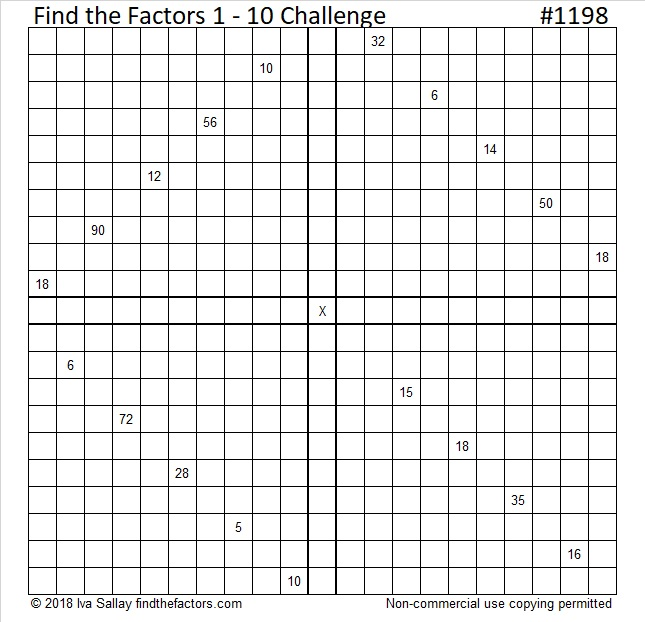Print the puzzles or type the solution in this excel file: 12 factors 1187-1198

Now I’ll share some facts about the number 1198:

• 1198 is a composite number.
• Prime factorization: 1198 = 2 × 599
• The exponents in the prime factorization are 1 and 1. Adding one to each and multiplying we get (1 + 1)(1 + 1) = 2 × 2 = 4. Therefore 1198 has exactly 4 factors.
• Factors of 1198: 1, 2, 599, 1198
• Factor pairs: 1198 = 1 × 1198 or 2 × 599
• 1198 has no square factors that allow its square root to be simplified. √1198 ≈ 34.612141198 is also palindrome 262 in BASE 23

# 1186 Challenge Puzzle

It shouldn’t be too hard to make your first move in this puzzle. After that, I don’t make any guarantees. You just need to write each number from 1 to 10 in each of the four boldly outlined areas so that the given clues are the products of the factors you wrote. Use logic to find all the factors and have fun doing it!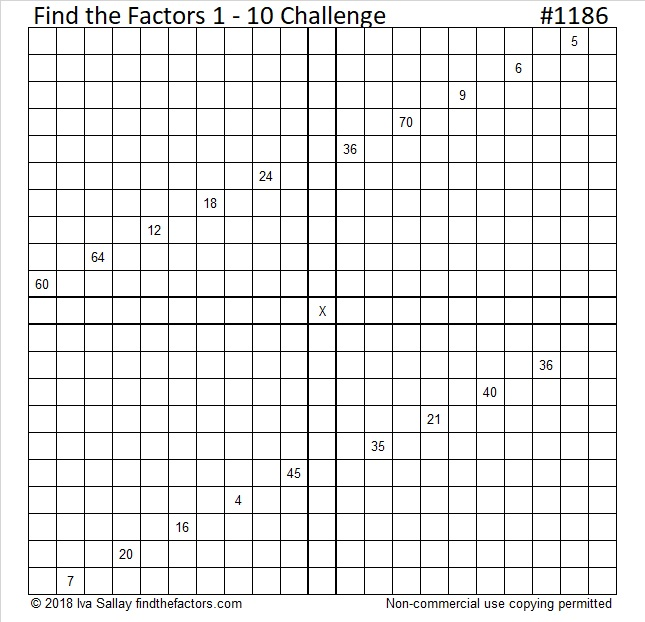Print the puzzles or type the solution in this excel file: 10-factors-1174-1186

What have I found out about the number 1186?

• 1186 is a composite number.
• Prime factorization: 1186 = 2 × 593
• The exponents in the prime factorization are 1 and 1. Adding one to each and multiplying we get (1 + 1)(1 + 1) = 2 × 2 = 4. Therefore 1186 has exactly 4 factors.
• Factors of 1186: 1, 2, 593, 1186
• Factor pairs: 1186 = 1 × 1186 or 2 × 593
• 1186 has no square factors that allow its square root to be simplified. √1186 ≈ 34.4383531² + 15² = 1186

1186 is the hypotenuse of a Pythagorean triple:
736-930-1186 calculated from 31² – 15², 2(31)(15), 31² + 15²

1186 is palindrome 989 in BASE 11

# 1173 Challenge Puzzle

Getting started on this Challenge Puzzle will take some thinking, but solving it is worth all the effort. Remember use logic, not guess and check, and you will eventually be successful!Print the puzzles or type the solution in this excel file: 12 factors 1161-1173

Here’s some information about the number 1173:

• 1173 is a composite number.
• Prime factorization: 1173 = 3 × 17 × 23
• The exponents in the prime factorization are 1, 1, and 1. Adding one to each and multiplying we get (1 + 1)(1 + 1)(1 + 1) = 2 × 2 × 2 = 8. Therefore 1173 has exactly 8 factors.
• Factors of 1173: 1, 3, 17, 23, 51, 69, 391, 1173
• Factor pairs: 1173 = 1 × 1173, 3 × 391, 17 × 69, or 23 × 51
• 1173 has no square factors that allow its square root to be simplified. √1173 ≈ 34.249091173 is the hypotenuse of a Pythagorean triple:
552-1035-1173 which is (8-15-17) times 69

1173 is palindrome 3B3 in BASE 18 (B is 11 base 10)
because 3(18²) + 11(18) + 3(1) = 1173

# 1160 Find the Factors Challenge

I love this particular puzzle. I had so much fun figuring out the logic. It’s a little bit complicated, but once you figure out the first move, it shouldn’t take too long to figure out most of the rest of the puzzle. Do give it a try!Print the puzzles or type the solution in this excel file: 10-factors-1148-1160

Here are some facts about the number 1160:

• 1160 is a composite number.
• Prime factorization: 1160 = 2 × 2 × 2 × 5 × 29, which can be written 1160 = 2³ × 5 × 29
• The exponents in the prime factorization are 3, 1, and 1. Adding one to each and multiplying we get (3 + 1)(1 + 1)(1 + 1) = 4 × 2 × 2 = 16. Therefore 1160 has exactly 16 factors.
• Factors of 1160: 1, 2, 4, 5, 8, 10, 20, 29, 40, 58, 116, 145, 232, 290, 580, 1160
• Factor pairs: 1160 = 1 × 1160, 2 × 580, 4 × 290, 5 × 232, 8 × 145, 10 × 116, 20 × 58, or 29 × 40
• Taking the factor pair with the largest square number factor, we get √1160 = (√4)(√290) = 2√290 ≈ 34.0587726² + 22² = 1160
34² +  2² = 1160

1160 is the hypotenuse of FOUR Pythagorean triples:
136-1152-1160 which is 8 times (17-144-145)
(It can also be calculated from 2(34)(2), 34² –  2², 34² +  2²)
192-1144-1160 which is 8 times (24-143-145)
(It can also be calculated from 26² – 22², 2(26)(22), 26² + 22²)
696-928-1160 which is (3-4-5) times 232
800-840-1160 which is (20-21-29) times 40

1160 is a palindrome when it is written in these three bases:
It’s 808 in BASE 12 because 8(12²) + 8(1) = 8(145) = 1160,
525 in BASE 15 because 5(15²) + 2(15) + 5(1) = 1160, and
404 in BASE 17 because 4(17²) + 4(1) = 4(290) = 1160

# 1147 Dragonfly Challenge

This “dragonfly” challenges you to solve the puzzle. It won’t be easy, but if you stick to using logic the entire time, you will be able to do it!Print the puzzles or type the solution in this excel file: 12 factors 1134-1147

Here are a few facts about the number 1147:

• 1147 is a composite number.
• Prime factorization: 1147 = 31 × 37
• The exponents in the prime factorization are 1 and 1. Adding one to each and multiplying we get (1 + 1)(1 + 1) = 2 × 2 = 4. Therefore 1147 has exactly 4 factors.
• Factors of 1147: 1, 31, 37, 1147
• Factor pairs: 1147 = 1 × 1147 or 31 × 37
• 1147 has no square factors that allow its square root to be simplified. √1147 ≈ 33.86739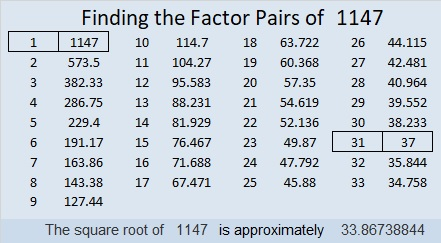1147 is the hypotenuse of a Pythagorean triple:
372-1085-1147 which is 31 times (12-35-37)

34² – 3² = 1147, so we are only 9 numbers away from the next perfect square.

1147 is a leg in a few Pythagorean triples including this primitive:
204-1147-1165 calculated from 2(34)(3), 34² – 3², 34² + 3²

I like the way 1147 looks when it is written in some other bases:
It’s 5151 in BASE 6 because 5(6³) + 1(6²) + 5(6) + 1(1) = 1147,
7B7 in BASE 12 (B is 11 base 10) because 7(12²) + 11(12) + 7(1) = 1147,
and VV in BASE 36 (V is 31 base 10) because 31(36) + 31(1) = 31(37) = 1147

# 1133 A Challenge Puzzle

I haven’t published a Find the Factors 1 – 10 Challenge puzzle for a while. Getting started on this one shouldn’t be difficult. The challenge will be in finishing it! Don’t guess and check. It can all be done using logic.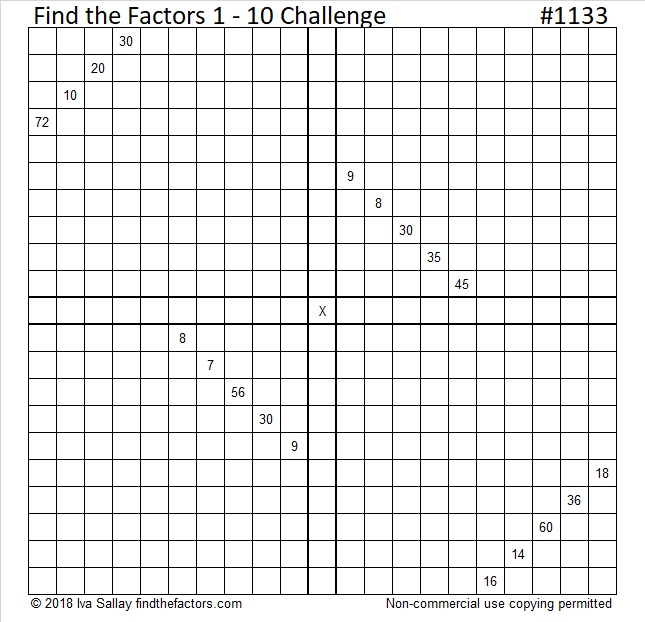Print the puzzles or type the solution in this excel file: 10-factors-1121-1133

Here are some facts about the number 1133:

Since both 11 and 33 are divisible by 11, we know 1133 can be evenly divided by 11.

• 1133 is a composite number.
• Prime factorization: 1133 = 11 × 103
• The exponents in the prime factorization are 1 and 1. Adding one to each and multiplying we get (1 + 1)(1 + 1) = 2 × 2 = 4. Therefore 1133 has exactly 4 factors.
• Factors of 1133: 1, 11, 103, 1133
• Factor pairs: 1133 = 1 × 1133 or 11 × 103
• 1133 has no square factors that allow its square root to be simplified. √1133 ≈ 33.66007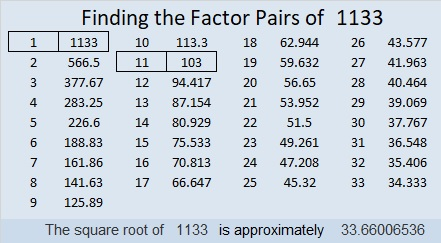1133 is the sum of the twenty-one prime numbers from 13 to 101.
1133 is also the sum of the fifteen prime numbers from 43 to 107.

# 1072 Find the Factors Challenge

These Find the Factors Challenge puzzles are tougher than my other puzzles, but they can still be solved using logic and basic multiplication and division facts. Go ahead, give it a try! I sincerely hope you will succeed!Print the puzzles or type the solution in this excel file: 12 factors 1063-1072

Now I’ll share some facts about the number 1072:

• 1072 is a composite number.
• Prime factorization: 1072 = 2 × 2 × 2 × 2 × 67, which can be written 1072 = 2⁴ × 67
• The exponents in the prime factorization are 4 and 1. Adding one to each and multiplying we get (4 + 1)(1 + 1) = 5 × 2 = 10. Therefore 1072 has exactly 10 factors.
• Factors of 1072: 1, 2, 4, 8, 16, 67, 134, 268, 536, 1072
• Factor pairs: 1072 = 1 × 1072, 2 × 536, 4 × 268, 8 × 134, or 16 × 67
• Taking the factor pair with the largest square number factor, we get √1072 = (√16)(√67) = 4√67 ≈ 32.74141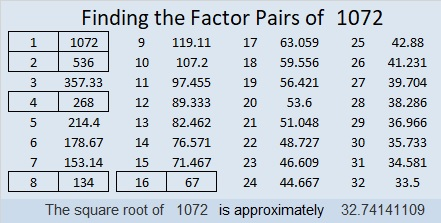1072 is also palindrome 646 in BASE 13 because 6(13²) + 4(13) + 6(1) = 1072

# 1060 A Challenge for Justin’s Birthday

Justin had no problems solving the puzzle I made for his last birthday, so this year I’ve made it tougher. Write the numbers from 1 to 10 in each of the four purple sections of the puzzle so that the clues are the products of the corresponding factors. Like always, there is only one solution. This puzzle can still be solved entirely by using logic and knowledge of basic multiplication and division facts. Will Justin be able to figure it out? I’m anxious to find out. Happy birthday, Justin!Here’s the same puzzle without added color if that’s better for printing.Print the puzzles or type the solution in this excel file: 10-factors-1054-1062

Now here are some things I’ve learned about the number 1060:

• 1060 is a composite number.
• Prime factorization: 1060 = 2 × 2 × 5 × 53, which can be written 1060 = 2² × 5 × 53
• The exponents in the prime factorization are 2, 1, and 1. Adding one to each and multiplying we get (2 + 1)(1 + 1)(1 + 1) = 3 × 2 × 2 = 12. Therefore 1060 has exactly 12 factors.
• Factors of 1060: 1, 2, 4, 5, 10, 20, 53, 106, 212, 265, 530, 1060
• Factor pairs: 1060 = 1 × 1060, 2 × 530, 4 × 265, 5 × 212, 10 × 106, or 20 × 53,
• Taking the factor pair with the largest square number factor, we get √1060 = (√4)(√265) = 2√265 ≈ 32.557641060 is the sum of consecutive prime numbers three different ways:
It is the sum of the prime numbers from 2 to 97, that’s all the prime numbers less than 100.
83 + 89 + 97 + 101 + 103 + 107 + 109 + 113 + 127 + 131 = 1060; that’s 10 consecutive primes
257 + 263 + 269 + 277 = 1060; that’s 4 consecutive primes

32² + 6² = 1060
24² + 22² = 1060

1060 is the hypotenuse of FOUR Pythagorean triples:
92-1056-1060
560-900-1060
636-848-1060
384-988-1060

1060 is a palindrome in some other bases:
884 in BASE 11
424 in BASE 16
202 in BASE 23

# 1053 Find the Factors Challenge

If you can multiply and divide and THINK, then you can solve this puzzle. Go ahead. Give it a try! To solve it, write the numbers from 1 to 10 in each of the four bold areas on the puzzle so that the given clues will be the products of those corresponding factors. Good luck!Print the puzzles or type the solution in this excel file: 12 factors 1044-1053

What can I tell you about the number 1053?

• 1053 is a composite number.
• Prime factorization: 1053 = 3 × 3 × 3 × 3 × 13, which can be written 1053 = 3⁴ × 13
• The exponents in the prime factorization are 4 and 1. Adding one to each and multiplying we get (4 + 1)(1 + 1) = 5 × 2 = 10. Therefore 1053 has exactly 10 factors.
• Factors of 1053: 1, 3, 9, 13, 27, 39, 81, 117, 351, 1053
• Factor pairs: 1053 = 1 × 1053, 3 × 351, 9 × 117, 13 × 81, or 27 × 39
• Taking the factor pair with the largest square number factor, we get √1053 = (√81)(√13) = 9√13 ≈ 32.4499627² + 18²  = 1053 so 1053 is the hypotenuse of a Pythagorean triple:
405-972-1053 calculated from 27² – 18², 2(27)(18), 27² + 18²

1053 is the sum of three consecutive powers of 3:
3 + 3 + 3 = 1053

1053 is 3033 in BASE 7 because 3(7³ + 7¹ + 7º) = 3(351) = 1053, and
it’s palindrome 878 in BASE 11 because 8(121) + 7(11) + 8(1) = 1053

# 1043 Find the Factors Challenge Puzzle

I made this particular Find the Factors 1-10 Challenge puzzle three weeks ago. It took me just under 30 minutes to solve it when I tried it again before publishing it. How long will it take you to solve it?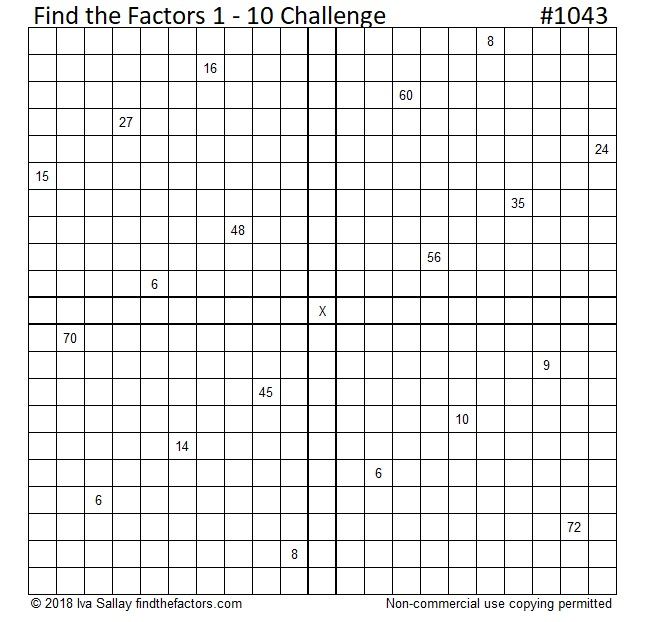Print the puzzles or type the solution in this excel file: 10-factors-1035-1043

Now here’s a little about the number 1043:

• 1043 is a composite number.
• Prime factorization: 1043 = 7 × 149
• The exponents in the prime factorization are 1 and 1. Adding one to each and multiplying we get (1 + 1)(1 + 1) = 2 × 2 = 4. Therefore 1043 has exactly 4 factors.
• Factors of 1043: 1, 7, 149, 1043
• Factor pairs: 1043 = 1 × 1043 or 7 × 149
• 1043 has no square factors that allow its square root to be simplified. √1043 ≈ 32.295511043 is the sum of consecutive prime numbers two different ways:
It’s the sum of the 21 prime numbers from 11 to 97 and,
it’s the sum of the 13 prime numbers from 53 to 107.

1043 is also the hypotenuse of a Pythagorean triple:
357-980-1043 which is 7 times (51-140-149)